/

### Constrained Quadratic Optimisation: 2. Solving the canonical problem

Next page

2.            Solving the canonical problem

We can solve the canonical problem using a variant of the Simplex algorithm, as follows:

(a)    We use the method of Lagrange multipliers, where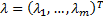and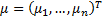are the Lagrange multipliers corresponding to the two sets of constraints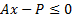and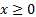respectively.

(b)   We introduce further ‘slack’ variables,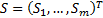for the constraints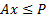, i.e. we define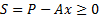.

(c)    Application of the Kuhn-Tucker conditions then implies that the canonical problem can be solved using a variant of the Simplex algorithm, using a Simplex ‘tableau’ (bearing in mind that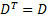) as follows, see e.g. Taha (1976):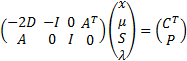subject to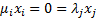for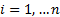,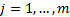and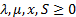.

Loosely speaking, the algorithm works as follows:

(i)      We start with a feasible solution (i.e. a solution that satisfies all of the constraints), which will be characterised by some value of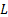;

(ii)    We then identify a methodical way of improving on(if possible), whilst staying within the set of feasible solutions; and

(iii)   We iterate (ii) until we run out of ability to improve.

Conveniently, because of the convex nature of the feasible solution set, the Simplex algorithm is in this case guaranteed to converge. Moreover, because the loss function is quadratic and positive definite, it converges to the global optimum and not just a local optimum.

The relative ease with which it is possible to solve quadratic optimisation problems is one reason why quadratic utility functions are so commonly used in quantitative finance, see Kemp (2010).

However, there is one complication. For the algorithm to work properly we need to start with a ‘feasible’ solution – if we start with one that isn’t feasible then the algorithm sometimes converges to a feasible solution but sometimes it doesn’t. We do not necessarily end up with a feasible solution merely by starting with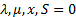, since the left hand side of the tableau then equates to zero, but the right hand side doesn’t. What we therefore need to do is to add additional variables to create a superset of the original optimisation problem for which it is easy to identify a (basic) feasible solution, the additional variables being set up in such a way that when we have converged on the solution to the superset problem we will then either:

(1)    Have reached a feasible solution to the original problem, in which case we have the solution; or

(2)    We still have an infeasible solution, in which case we can conclude that there was no feasible solution to the original problem.